# Functions | Concept Booster, IIT JEE, Class 12 notes

• 2
•
• 4
•
• 2
•
•
•
8
Shares

Know Functions, Types of Functions, What is Composite Function ? Periodic Functions ? Properties of functions. You should learn set theory and relations before learning about functions.

# Functions

A function defined from set A to set B is a special case of a relation defined from set A to set B.

Let A and B be two non empty sets then a function defined from set A to B is a rule that each element of set A is uniquely associated with a element of set B.

## Domain Co-domain and Range of a function

Let’s consider a function from set a to b as shown in figure.

• Set A is called domain of the function.
• Set B is called Co-domain of the function.
• Set of elements of set B which are associated with elements of set a is called Range of the function.

#### Image and Pre image

In the above example
A is called as the image of 1. And 1 is called as Pre-image of a.
Similarly
B is image of 2 and 2 is pre- image of B.

Note: if there exists any vertical line on the graph of function which can cut the graph of function at more than one point, then it means that for a particular x we got more than one value of y hence by definition it’s not a function .

### Domain

Talking for a function y= f(x)

X is the input and f is a rule as per which we get the value of function i.e Y.

• X is input of function and is called as independent variable.
• Y is output of function and is called as dependent variable.

Domain of a function y = f(x) is the set of values of x for which the function is defined.

Note:
if domain of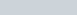and domain of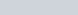then
• Domain of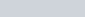is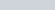• Domain of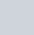is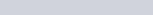• Domain of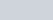is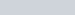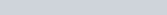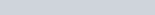• Domain of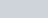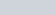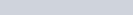• Domain of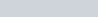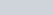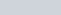### Range

For a function y = f(x), range is the set of values which the function attains for all the inputs within its domain, means range is the set of values of “y”.

#### Ways to determine range of a function

• if possible then express x in terms of y. i.eand find the domain of this function i.e value of y for which this function is defined,by using methods we studied above.
This will be the required range as we obtain set of values of “y”.
• by graph
If we plot the graph of the function or the graph is given to us then simply we had to see the set of values of y , hence range can be found.

• Using Monotonocity

(1) if a function is continuous i.e it’s graph can be drawn without lifting off pen from the paper. And it’s monotonic increasing for interval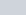i.e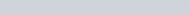Note- equality should hold at discrete points in above expression then the function will be monotonic increasing. Similarly we can state for monotonic Decreasing.

Then Range is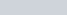(2) if function is continuous and monotonic decreasing inThen Range is(3) if f(x) is continuous in [a,b] and f`(x)= 0 has a solutionThen Range is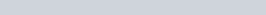#### Points to note :

• If f(x) is a odd degree polynomial defined for all the numbers on real line. Then its range will be• Range of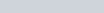is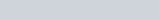## Equal/Identical Functions

Two functions f(x) and g(x) are defined to be equal/identical functions if
(I) Domain of f(x) = Domain of g(x)
(II) Range of f(x) = Range of g(x)
(III) f(x) = g(x)
All these points should hold for all values of “x” in their common domain.

## Types of Functions

### One – One function

Any function f(x) from set A to set B is defined to be one one function if different elements of set A have different images in set B. This Mapping is also Known as INJECTIVE MAPPING.

### Many- One function

Any function f(x) from set A to B is defined as many – one if
Atleast there are atleast two elements of set A which have same images in set B.

### Onto function

A function f(x) from set A to B is called as onto function if
Every element of set B has atleast one Pre-image in set A i.e every element of set b must be associated. Here we can see that
Range of function = Co-domain for a onto function. This is also known as surjective Mapping.

### Into Function

For a function from set A to B. If there exists atleast one element of set B which has no Pre-image in set A. This function is called as Into Function.
Here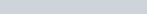### Bijective Function

A function which is both one-one and onto is called as bijective function.

# Even and Odd Functions

if for a function y = f(x)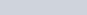Then function is defined to be a even function. For example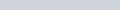are even functions.
Similarly if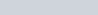Then the function is defined to be a odd function.for example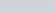is a odd function.

## Properties

• if we plot the graph of a even function then we can observe that the graph of a even function is symmetric about the Y axis. As in case of graph of cos(x).
• if we plot the graph of odd functions then we can see a different type of symmetry said as symmetry about a point. The graph of all of functions are symmetric about origin.
• derivative of an Even function is a odd function and vice versa.
• f(x) = 0 is the only function which is both even and odd together, we can justify it by the definitions of even and odd function.

## Composite FunctionsLet’s take the situation that, we have a function “f” from set X to set Y, also we are having another function “g” from set Y to set Z. Now we can combine these two functions f and g and we can get a composite Function g(f(x)) from set X to set Z.
Denoted as “gof” is a composite Function from set X to set Z. Now let’s analyse it through the mapping shown in above figure.
If we enter “a” as input to g(f(x)) the value/output we obtain will be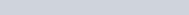Similarly we can define f(g(x)) i.e fog.

Note:

1.for gof to be defined the range of f(x) should be in domain of g(x) i.e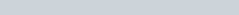2. In general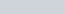# Periodic Functions

If the graph of a functions repeats after a finite interval then the function is called as periodic function.

The length of this interval is known as period of the function, while the smallest possible interval after which graph repeats is fundamental proof of function.
Mathematically, a function f(x) is periodic if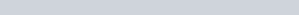### Properties of Periodic Functions

Here we will state some of the results and properties without proof:
• if f(x) has period “T”, then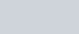will all have same period T.
• if f(x) has period “T” , then the period of f(ax+b) will be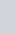.
• if period of f(x) is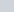and period of g(x) is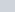then the period of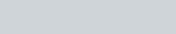Will beif this L.C.M doesn’t exist then the resultant functions won’t be periodic.
We have to check seperately whether it’s fundamental period of not.
• All constant functions are periodic as their graph repeats but they have no fundamental period.
• if “gof” is defined and f(x) have period “T”, then gof will also have period “T”. For fundamental period we have to check it.

Grasped Concepts of Functions ? Time to learn Inverse Trigonometric Functions